A monochromatic beam of light falls on YDSE apparatus at some angle (say θ) as shown in figure. A thin sheet of glass is inserted in front of the lower slit S2. The central bright fringe (path difference = 0) will be obtained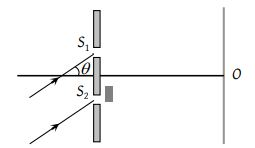(1) At O

(2) Above O

(3) Below O

(4) Anywhere depending on angle θ, thickness of plate t and refractive index of glass μ

Concept Questions :-

Young Double slit experiment
High Yielding Test Series + Question Bank - NEET 2020

Difficulty Level:

Two ideal slits S1 and S2 are at a distance d apart, and illuminated by light of wavelength λ passing through an ideal source slit S placed on the line through S2 as shown. The distance between the planes of slits and the source slit is D. A screen is held at a distance D from the plane of the slits. The minimum value of d for which there is darkness at O is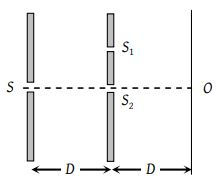(1) $\sqrt{\frac{3\lambda D}{2}}$

(2) $\sqrt{\lambda D}$

(3) $\sqrt{\frac{\lambda D}{2}}$

(4) $\sqrt{3\lambda D}$

Concept Questions :-

Young Double slit experiment
High Yielding Test Series + Question Bank - NEET 2020

Difficulty Level:

Two point sources X and Y emit waves of same frequency and speed but Y lags in phase behind X by 2πl radian. If there is a maximum in direction D the distance XO using n as an integer is given by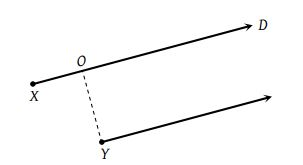(1) $\frac{\lambda }{2}\left(n-l\right)$

(2) $\lambda \left(n+l\right)$

(3) $\frac{\lambda }{2}\left(n+l\right)$

(4) $\lambda \left(n-l\right)$

Concept Questions :-

Diffraction
High Yielding Test Series + Question Bank - NEET 2020

Difficulty Level:

A beam with wavelength λ falls on a stack of partially reflecting planes with separation d. The angle θ that the beam should make with the planes so that the beams reflected from successive planes may interfere constructively is (where n =1, 2, ……)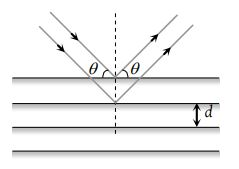(1) ${\mathrm{sin}}^{-1}\left(\frac{n\lambda }{d}\right)$

(2) ${\mathrm{tan}}^{-1}\left(\frac{n\lambda }{d}\right)$

(3) ${\mathrm{sin}}^{-1}\left(\frac{n\lambda }{2d}\right)$

(4) ${\mathrm{cos}}^{-1}\left(\frac{n\lambda }{2d}\right)$

Concept Questions :-

Interference vs Diffraction
High Yielding Test Series + Question Bank - NEET 2020

Difficulty Level:

Two coherent sources separated by distance d are radiating in phase having wavelength λ. A detector moves in a big circle around the two sources in the plane of the two sources. The angular position of n = 4 interference maxima is given as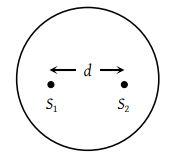(1) ${\mathrm{sin}}^{-1}\frac{n\lambda }{d}$

(2) ${\mathrm{cos}}^{-1}\frac{4\lambda }{d}$

(3) ${\mathrm{tan}}^{-1}\frac{d}{4\lambda }$

(4) ${\mathrm{cos}}^{-1}\frac{\lambda }{4d}$

Concept Questions :-

Interference vs Diffraction
High Yielding Test Series + Question Bank - NEET 2020

Difficulty Level:

A circular disc is placed in front of a narrow source. When the point of observation is 2 m from the disc, then it covers first HPZ. The intensity at this point is I. When the point of observation is 25 cm from the disc then intensity will be

(1) ${\left(\frac{{R}_{6}}{{R}_{2}}\right)}^{2}I$

(2) ${\left(\frac{{R}_{7}}{{R}_{2}}\right)}^{2}I$

(3) ${\left(\frac{{R}_{8}}{{R}_{2}}\right)}^{2}I$

(4) ${\left(\frac{{R}_{9}}{{R}_{2}}\right)}^{2}I$

Concept Questions :-

Interference vs Diffraction
High Yielding Test Series + Question Bank - NEET 2020

Difficulty Level:

In a single slit diffraction of light of wavelength λ by a slit of width e, the size of the central maximum on a screen at a distance b is

(1) $2b\lambda +e$

(2) $\frac{2b\lambda }{e}$

(3) $\frac{2b\lambda }{e}+e$

(4) $\frac{2b\lambda }{e}-e$

Concept Questions :-

Diffraction
High Yielding Test Series + Question Bank - NEET 2020

Difficulty Level:

The ratio of intensities of consecutive maxima in the diffraction pattern due to a single slit is

(1) 1 : 4 : 9

(2) 1 : 2 : 3

(3) $1\text{\hspace{0.17em}}:\frac{4}{9\text{\hspace{0.17em}}{\pi }^{2}}:\frac{4}{25\text{\hspace{0.17em}}{\pi }^{2}}$

(4) $1:\frac{1}{{\pi }^{2}}:\frac{9}{{\pi }^{2}}$

Concept Questions :-

Diffraction
High Yielding Test Series + Question Bank - NEET 2020

Difficulty Level:

In a YDSE bi-chromatic light of wavelengths, 400 nm and 560 nm are used. The distance between the slits is 0.1 mm and the distance between the plane of the slits and the screen is 1m. The minimum distance between two successive regions of complete darkness is

(1) 4 mm

(2) 5.6 mm

(3) 14 mm

(4) 28 mm

Concept Questions :-

Young Double slit experiment
High Yielding Test Series + Question Bank - NEET 2020

Difficulty Level:

In Young's double-slit experiment, the intensity at a point is (1/4) of the maximum intensity. Angular position of this point is :

(1) sin-1(λ/d)

(2) sin-1(λ/2d)

(3) sin-1(λ/3d)

(4) sin-1(λ/4d)

Concept Questions :-

Young Double slit experiment